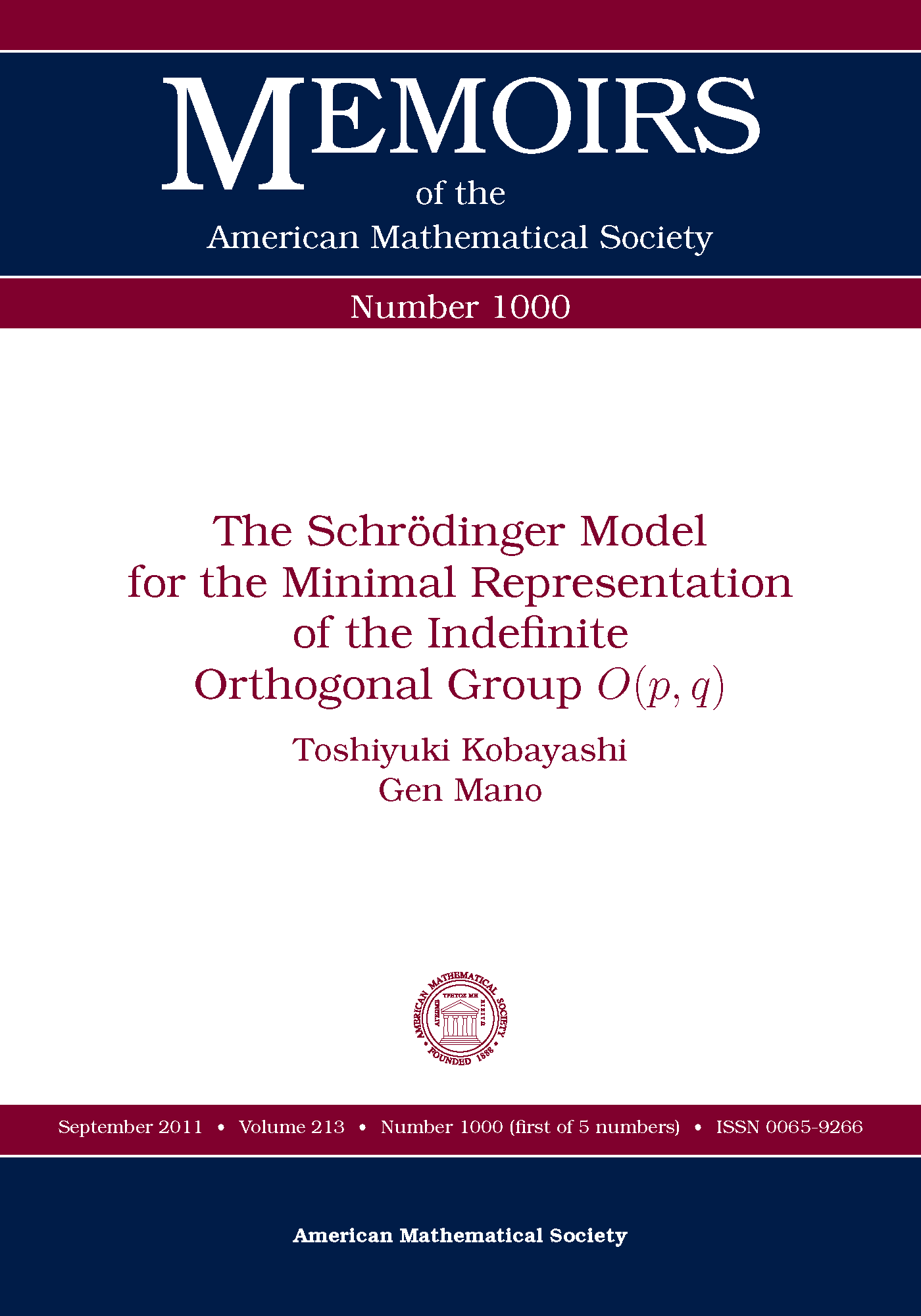## T. Kobayashi and G. Mano, The Schrödinger model for the minimal representation of the indefinite orthogonal group O(p, q), Mem. Amer. Math. Soc. 212, no. 1000, 2011, vi+132 pp., (accepted for publication on 31 July, 2008; published online first, on 4 February 2011). DOI: 10.1090/S0065-9266-2011-00592-7. arXiv:0712.1769 [math.RT]..

We introduce the 'Fourier transform' FC on the isotropic cone C associated to an indefinite quadratic form of signature (n1,n2) on Rn (n=n1+n2: even). This transform is in some sense the unique and natural unitary operator on L2(C), as is the case with the Euclidean Fourier transform FR^n on L2(Rn). Inspired by recent developments of algebraic representation theory of reductive groups, we shed new light on classical analysis on the one hand, and give the global formulas for the L2-model of the minimal representation of the simple Lie group G=O(n1+1,n2+1) on the other hand.

The transform FC expands functions on C into joint eigenfunctions of fundamental differential operators which are mutually commuting, self-adjoint, and of second order. We decompose FC into the singular Radon transform and the Mellin-Barnes integral, find its distribution kernel, and establish the inversion and the Plancherel formula. The transform FC reduces to the Hankel transform if G is O(n,2) or O(3,3) ≈ SL(4,R).

The unitary operator FC together with multiplications and translations coming from the conformal transformation group CO(n1,n2)Rn_1+n_2 generates the minimal representation of the indefinite orthogonal group G. Various different models of the same representation have been constructed by Kazhdan, Kostant, Binegar-Zierau, Gross-Wallach, Zhu-Huang, Torasso, Brylinski, and Kobayashi-Ørsted, and others. Among them, our model gives the global formula of the whole group action on the simple Hilbert space L2(C), and generalizes the classic Schrödinger model L2(Rn) of the Weil representation. Here, FC plays a similar role to FR^n.

Yet another motif is special functions. Large group symmetries in the minimal representation yield functional equations of various special functions. We find explicit K-finite vectors on L2(C), and give a new proof of the Plancherel formula for Meijer's G-transforms.